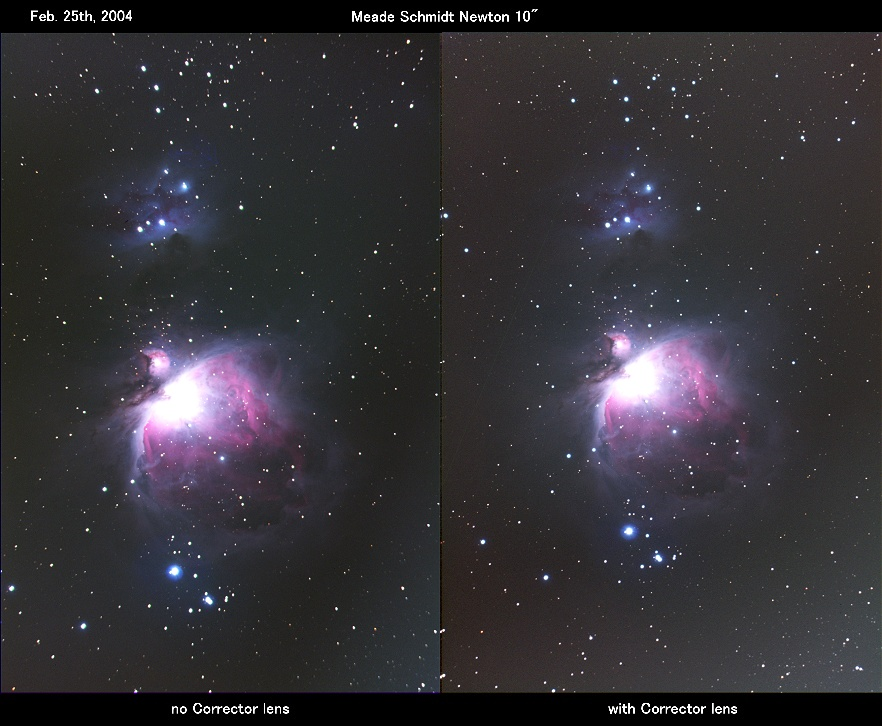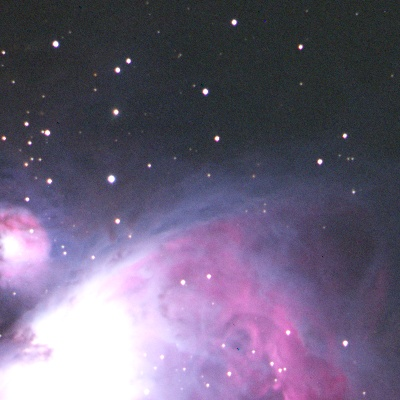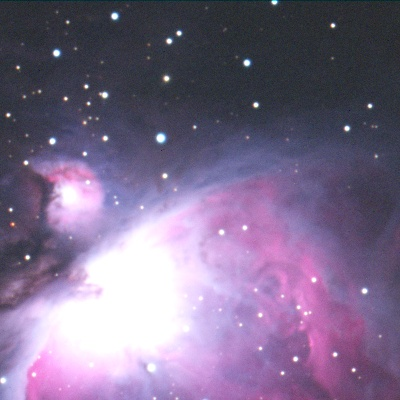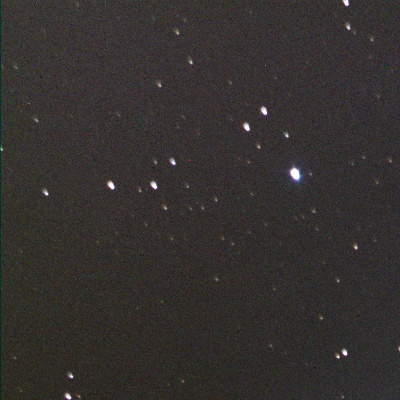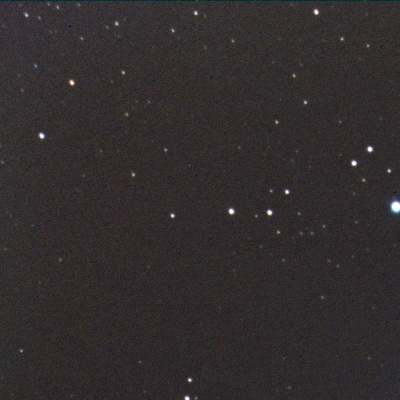T-Reducer for Schmidt Newton

The Newtonian telescope is very popular, but is known for its coma, and this coma makes for poor photography. The Schmidt Newtonian telescope is half that of Newtonian. However, the Schmidt Newtonian's coma is still large. The Maksutov Newtonian telescope has much lower coma than the Schmidt Newtonian, but the Meniscus lens is expensive.

Why is there no coma corrector for the Schmidt Newton like there is for the Newtonian? It should be easier to correct the coma of a Schmidt Newtonian than a Newtonian because of its lower coma.

A doublet lens seems to be best suited to work as a reducer for the Schmidt Newtonian I have named this device the "T-Reducer." I used a Edmund doublet lens, f.l.=500mm & D=50mm. It is best positioned 930mm away from the primary mirror of Schmidt Newtonian (D=10" Fno=4.0). Spot diagrams are very good forcomparing Newtonian, Maksutov and the original Schmidt Newtonian.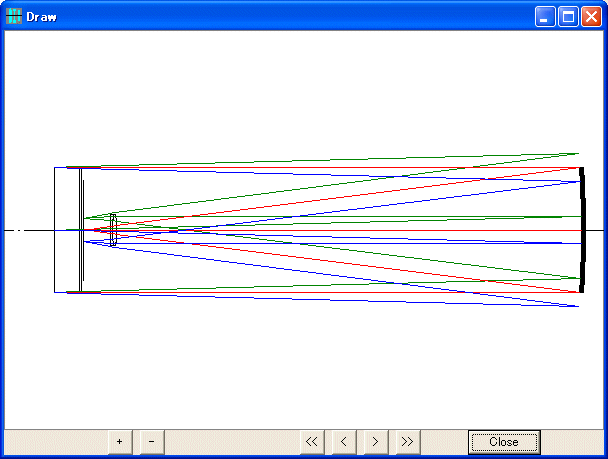Ray Diagram of Schmidt Newton telescope + T-Reducer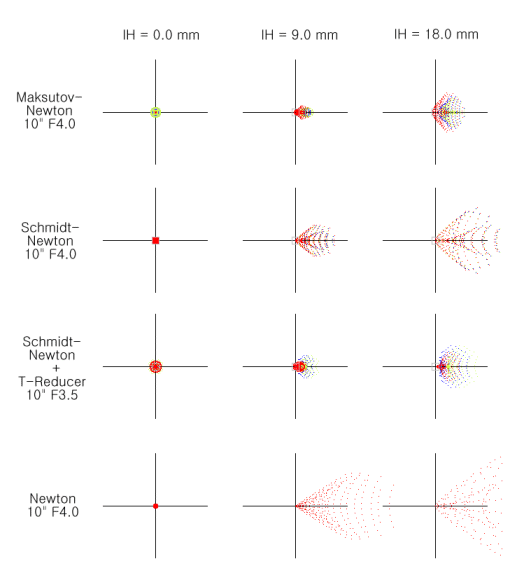Spot diagram

The T-Reducer produces a minor spherical abberation on the main optical axis, but other abberations are smaller than the other telescope designs. As the smaller spot diagram shows this spherical abberation is negligible. The Edmund doublet lens is achomatic. If an apochromatic lens is used, even better photographs could be produced.

Here is an apochromatic objective lens, Borg 50ED( f.l.=500mm), being applied as a coma corrector.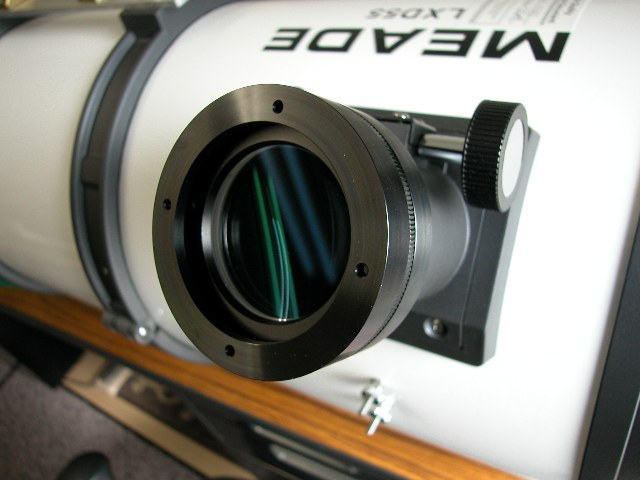Here then is the result. Note the reduced coma in the right image.